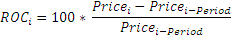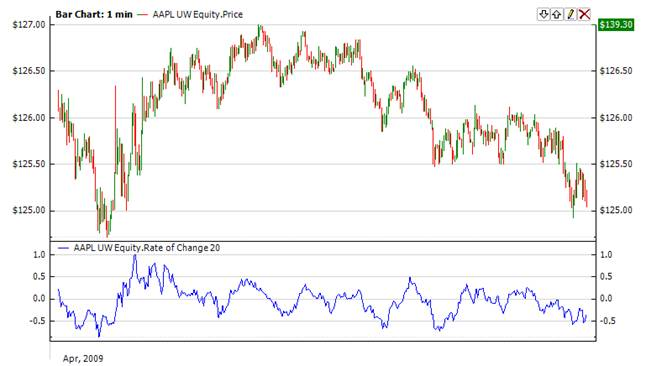﻿Rate Of Change

# Rate Of Change

The Rate of Change (Roc) function measures rate of change relative to previous periods.The function is used to determine how rapidly the data is changing. The factor of 100 is usually used to merely make the numbers easier to interpret or graph. The function can be used to measure the Roc of any data series, such as price or another indicator.Market Signals

The most popular way to use the indicator is as follows. When indicator is above the 0.5 line, it is a buy signal.  If indicator crosses the 0.5 line from above it is a sell signal.CalculationChart ExampleImplementation and Usage

To initialize Roc indicator use one of the following constructors:

Roc – sets default values: period = 14

Roc(Int32) – sets value for period

Roc(TimeSpan) – sets time period

Use

ROC - property to get current valueExample
C#
``` 1// Create new instance
2Roc roc = new Roc(28);
3
4// Number of stored values
5roc.HistoryCapacity = 2;
6